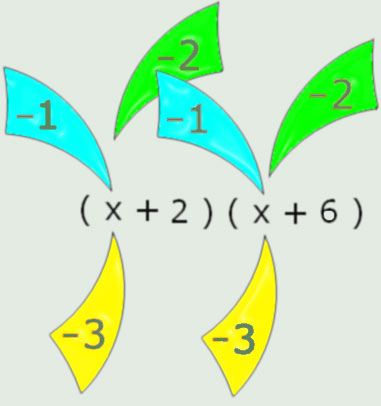#### You may also like### Converse

Clearly if a, b and c are the lengths of the sides of an equilateral triangle then a^2 + b^2 + c^2 = ab + bc + ca. Is the converse true?### Consecutive Squares

The squares of any 8 consecutive numbers can be arranged into two sets of four numbers with the same sum. True of false?### Parabolic Patterns

The illustration shows the graphs of fifteen functions. Two of them have equations y=x^2 and y=-(x-4)^2. Find the equations of all the other graphs.

# Minus One Two Three

##### Age 14 to 16Challenge Level

If you substitute $-1$, $-2$ or $-3$, into an algebraic expression you'll get three results. Is it possible to tell in advance which of those three substitutions will produce the greatest result and which will produce the least ?

Substitute $-1$, $-2$ and $-3$ into $(x + 2)(x + 6)$ here, and see what you get.Now can you predict for these ?